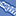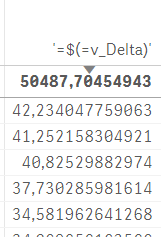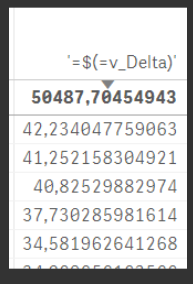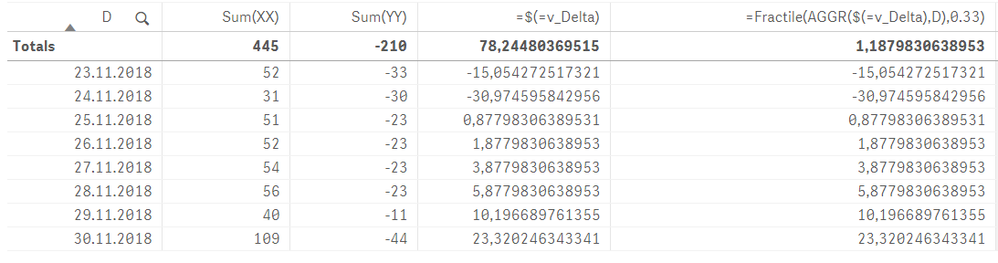# New to Qlik Sense

If you’re new to Qlik Sense, start with this Discussion Board and get up-to-speed quickly.

cancel
Showing results for
Did you mean:Partner

## Complex variable as Fractile source (chart function)

Hello how are you,

I made statistical variable "v_Delta" as :

`SUM(XX) - (LinEst_M(total XX, YY) * SUM(YY) + LinEst_B(total XX, YY))`

If I try to use it on KPI as:

`=Fractile(\$(=v_Delta), 0.33)`

It fails and shows no value.

V_Delta shows correct amounts if used in table chart, so that's not the problem:Also in expression editors variable shows value:Any ideas???

Labels (1)
• ### chart functions

1 Solution

Accepted SolutionsPartner
Author

I added granularity for the D filter in this qvf.

It seems that Fractile-function AND linest_m with total parameter need array of values to compute correctly.

9 RepliesMVP

What is your dimension when you use this in the chart? May be try this

```=Fractile(Aggr(
\$(=v_Delta)
, Dimension/s), 0.33)```Partner
Author
KPI has no dimensions only measuresMVP

Lol sure, but this chart does, does it not?Partner
Author

Same thing with AGGR-wrapping, shows only "-" as is its not valid expressionMVP

Would you be able to share a sample to check this out?Partner
Author

Here you go.MVP

So, if I don't filter on anything and use this expression

`Fractile(Aggr(\$(v_Delta), D), 0.33)`

I get thisBut you mentioned to filter on date, if I do that, then even the value within the chart goes awayWould you be able to point out what exactly are you hoping to get from the sample attached? Is it based on selection in Date? What is the expected output within KPI when you select 25.11.2018?Partner
Author

As you can see, every date (D) has incorrect calculation on fractile, it is just the v_delta, but total fractile is correct.

Why the v_Delta fails calculating with one D selection ?Partner
Author

I added granularity for the D filter in this qvf.

It seems that Fractile-function AND linest_m with total parameter need array of values to compute correctly.